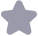### 数字信号处理课后习题答案(吴镇扬)收藏

(4)n(n)=mB( -1 令(m)=R(m),则x(z)=∑x 根据线性加权性质可得: H1()M(1-)-z(1-x) ≠0 I-F k -1 O)=∑m形=∑m=1+2+3+…+(M-1) M(M-1) 0 0 2 3.9图略 (n)=1(m)*(m)={12,3,4,5,3,1,-1,-3,-5,-4,-3-2,- 0(m)=(m)⑧(m)={-3,-1,,3,5,3,1,-1,-3,-5} 3.12 f DFTIx(n)cos(2r m/N)n)] -1 2丌m 2丌m x(n)[ e +e] hn n=0 -1 2丌m 2丌m 2兀h ∑x(m) / n=0 [(k-m)+x(k+m)] - 2丌m 2Th DFTn)sin((2m/M)n) ]=I( '-en le N 2 n=0 [r(k-m)-(+m) N-1 解:H()=∑m(n) 刀=0 H()=∑y(n)W"=∑(n)WM=∑m(m)W=x( n=0 n=0 刀=0 0≤h<M-1 314解:(h)={ xn)yrN 0 K()=∑n(n)WmM=∑1(")Wm=∑x(∥m n=0 刀=0,2r /0.1.2 ∑(n0≤k≤nN-1 ()0≤k≤M (h-M)N≤≤2-1 =((h)Rp(h) 互-(1-1)M(-1)≤k≤rM-1 3.15解:(1)根据DFT的共轭对称性有: r(k)=DFTRE(nl=-[F(K)+F(N-k) 11 1-b 1-b 21-ak 1-60) 1-aw? N-k 1-b N-k 1-b 1-b ]+[ 21-am b万 1aw 1-b 1-a, =∑a"WF / 1(n)=a"l(m) 同理:)=n1[(0)-F(M-,1-b b 2j 1-b在 1(m)=b(n) 3.15(2)()=1∴x(m)=(m) ()=M∴(n)=6(n) 3.18解:K()=DF7x(n)0≤k≤19 ()=DFL(n)]0≤k≤19 R(A)=(A)()0≤≤19,/(m)=DF[R)0≤k≤19 根据循环卷积定理可知/(n)=4(m)⑧1(m)(20点循环卷积); 但x(n)*1(n)不为零长度为27,所以1(m)*1(n)若以20为周期 进行延拓必然会产生7点的重叠; 因此,n(m)中7至19点与x(m)*1(n)相同。 319(1)周期卷积的主值序列为:/(mn)R(n)={63,6,10,1412,9} (2)循环卷积/(n)=6,3,6,10,1412,9}; (3)线性卷积为/(n)=1,3,6,10,14,12,9,5,0,0,0,0} 3.21按时间抽取算法:输出顺序, 输入倒序(0,8,4,12,2,10,6,14,1,9,5,13,3,11,7,15) 共有4(16=24)节 第一节:数据点间距、蝶形类型均是1, 所乘因子:0 第二节:数据点间距、蝶形类型均是2 所乘因子:W0,; 第三节:数据点间距、蝶形类型均是4 所乘因子:0,2,,W6 第四节:数据点间距、蝶形类型均是8 所乘因子:W0,W1,形2,n3,W4,,n6, 3.21按频率抽取算法:输入顺序, 输出倒序(0,8,4,12,2,10,6,14,1,9,5,13,3,11,7,15); 共有4(16-24)节 第一节:数据点间距、蝶形类型均是8, 所乘因子:W0,M,2,n3,4,W,6, 第二节:数据点间距、蝶形类型均是4, 所乘因子:M0,M2,W4,B; 第三节:数据点间距、蝶形类型均是2,所乘因子:丌0,W; 第四节:数据点间距、蝶形类型均是l,所乘因子:W°; 3.27解:从n)的长度为60,每段数据的长度为00,循环卷积的长度为28 所以根据重叠保留法可知相邻两段数据必有650-1)点重叠,在00点之 后须加28个零值点,所以卷积之后必须去掉前49点和危28点; 所以,N=49,M=128-49-28=51,取数的序号为49~99。 3.31解题思路: 2N-1 ∑(h) - 20 24 2 DH()2+∑()2N =0 h=w - 2 ∑A形+∑(+M)"令h=kM '=0 ∑[(A)+H2,M(h+M) 2=0 2 2N ∑[I(h)+(-1)I(h+M 2N =0 如为偶数2;则x(2)=∑可(句+(h+M)2 ∑[(h)+H(k+M =0 如n为奇数2x+1,则x(2+1)=∑{[h-(+M)2 =0 22[()-(+M)W,3

...展开详情

抢沙发
一个资源只可评论一次，评论内容不能少于5个字yj895551917

关注 私信 TA的资源

上传资源赚积分,得勋章
最新推荐数字信号处理课后习题答案(吴镇扬) 22积分/C币 立即下载
1/11试读已结束，剩余7页未读...

22积分/C币 立即下载 ＞